# Tubing Length Change due to Piston Effect

This article demonstrates a piston effect and how it will change the length of tubing with full detailed calculations.

For the analysis, Lubinski’s sign conventions are used.

Compression force = ⇑(+)

Tensile force = ⇓ (-)

Shorten in Length = (-)

Elongate in length = (+)

Note: The tubing is free to move.

Please see this in the reference section to get a full paper.

Total force change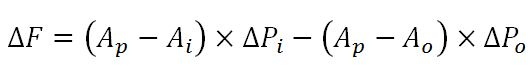Total length change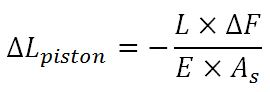Where;

Ai = Tubing ID area (in2)

Ao = Tubing OD area (in2)

Ap = Packer Seal Bore Area (in2)

As = Cross sectional area of tubing (in2)

L = Length of tubing (in)

ΔPo = Change in annulus pressure @ packer

ΔPi = Change in tubing pressure @ packer

ΔF =Change in force (lb)

ΔLpiston = Change in length due to piston effect (in)

E = Young modulus of steel (30×106 psi)

Pi = pressure in tubing (psi)

Po= pressure in annulus (psi)

These formulas are suitable for both configurations shown in Figure 1.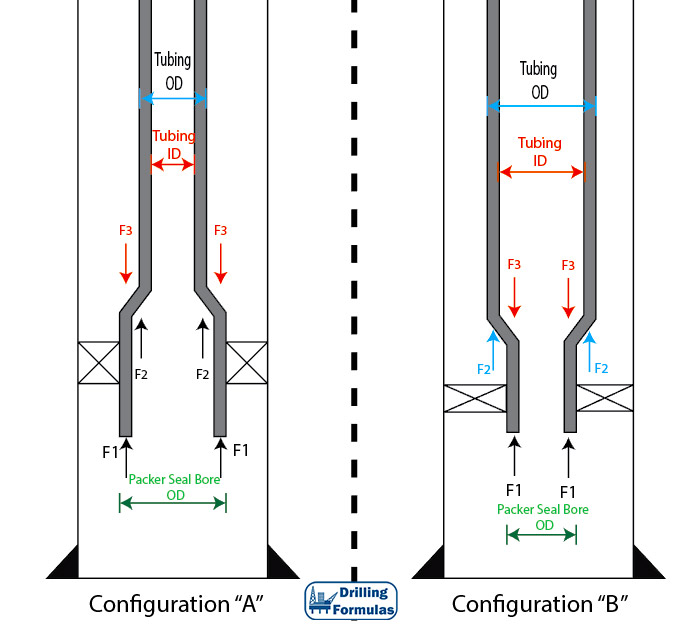Figure 1 – Packer Configuration

Example

Packer is set at 10,000 ft.

Tubing and packer are free to move.

The well is vertical.

4.5” Tubing

ID of tubing = 3.862”

Packer seal bore outside diameter = 5.0”

Weight per length = 17.7 lb/ft

Initial Condition

Fluid in annulus = 10.0 ppg

Fluid in tubing = 10.0 ppg

Tubing pressure = 0 psi

Annulus pressure = 0 psi

Final Condition

Fluid in annulus = 8.0 ppg

Fluid in tubing = 10.0 ppg

Tubing pressure = 1,500 psi

Annulus pressure = 0 psi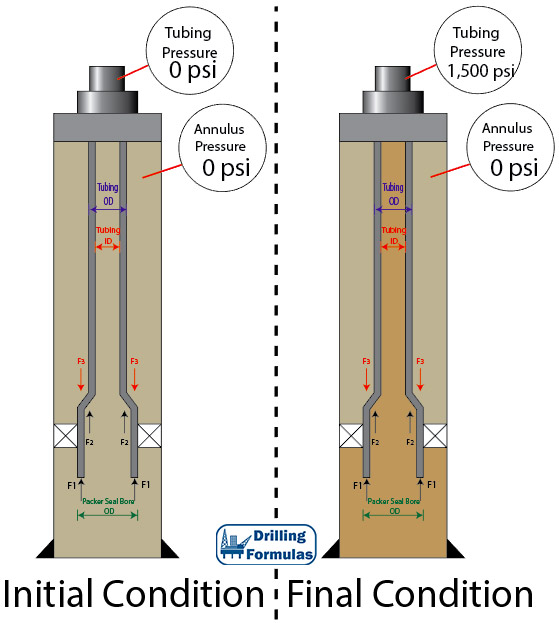Figure 2 – Initial and Final Condition

Calculate Areas

Ai = Tubing ID area (in2)

Ai= (π÷4) × 3.8622 = 11.497 in2

Ao = Tubing OD area (in2)

Ao= (π÷4) × 4.52 = 15.904 in2

Ap = Packer Seal Bore Area (in2)

Ap= (π÷4) × 5.02 = 19.635 in2

As = Cross sectional area of tubing (in2)

As = Ao – Ai = 15.904 – 11.497 = 4.407 in2

Determine Pressure at Packer at Initial Condition

Po @ surface = 0 psi

Pi @ surface = 0 psi

Po @ packer = Po @ surface + Hydrostatic P in annulus = 0 + (0.052×10×10,000) = 5,200 psi

Pi @ packer = Pi @ surface + Hydrostatic P in tubing = 0 + (0.052×10×10,000) = 5,200 psi

Determine Pressure at Packer at Final Condition

Po @ surface = 0 psi

Pi @ surface = 1500 psi

Po @ packer = Po @ surface + Hydrostatic P in annulus = 0 + (0.052×10×10,000) = 5,200 psi

Pi @ packer = Pi @ surface + Hydrostatic P in tubing =1,500 + (0.052×8×10,000) = 5,660 psi

Determine Pressure Change (ΔP)

ΔPo = Change in annulus pressure @ packer

ΔPo = 5,200 – 5,200 = 0 psi

ΔPi = Change in tubing pressure @ packer

ΔPi = 5,660 – 5,200 = 460 psi

Determine Force Change (ΔF)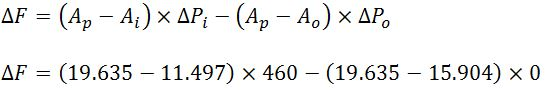ΔF = 3,744 lb (compression force (+))

Determine Length Change (ΔLpiston)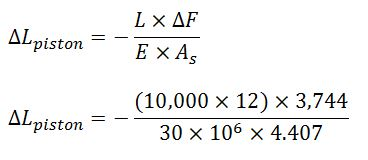ΔLpiston = – 3.4 inch (shorten)

Conclusion

Based on the given information, change in force acting at the packer is 3,744 lb (compression force (+)) and length change due to piston effect is 3.4 inch (shorten).

References

Jonathan Bellarby, 2009. Well Completion Design, Volume 56 (Developments in Petroleum Science). 1 Edition. Elsevier Science.

Wan Renpu, 2011. Advanced Well Completion Engineering, Third Edition. 3 Edition. Gulf Professional Publishing.

Ted G. Byrom, 2014. Casing and Liners for Drilling and Completion, Second Edition: Design and Application (Gulf Drilling Guides). 2 Edition. Gulf Professional Publishing.

Lubinski, A., & Althouse, W. S. (1962, June 1). Helical Buckling of Tubing Sealed in Packers. Society of Petroleum Engineers. doi:10.2118/178-PA

Share the joy
Tagged , , , . Bookmark the permalink.